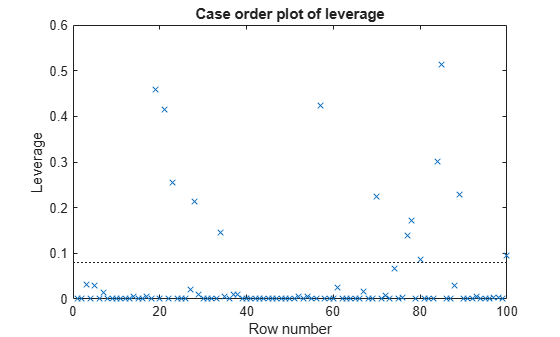Documentation

## Generalized Linear Model Workflow

This example shows how to fit a generalized linear model and analyze the results. A typical workflow involves the following: import data, fit a generalized linear model, test its quality, modify it to improve the quality, and make predictions based on the model. It computes the probability that a flower is in one of two classes, based on the Fisher iris data.

### Step 1. Load the data.

Load the Fisher iris data. Extract the rows that have classification versicolor or virginica. These are rows 51 to 150. Create logical response variables that are `true` for versicolor flowers.

```load fisheriris X = meas(51:end,:); % versicolor and virginica y = strcmp('versicolor',species(51:end));```

### Step 2. Fit a generalized linear model.

Fit a binomial generalized linear model to the data.

```mdl = fitglm(X,y,'linear',... 'distr','binomial')```
```mdl = Generalized linear regression model: logit(y) ~ 1 + x1 + x2 + x3 + x4 Distribution = Binomial Estimated Coefficients: Estimate SE tStat pValue ________ ______ _______ ________ (Intercept) 42.638 25.708 1.6586 0.097204 x1 2.4652 2.3943 1.0296 0.30319 x2 6.6809 4.4796 1.4914 0.13585 x3 -9.4294 4.7372 -1.9905 0.046537 x4 -18.286 9.7426 -1.8769 0.060529 100 observations, 95 error degrees of freedom Dispersion: 1 Chi^2-statistic vs. constant model: 127, p-value = 1.95e-26 ```

### Step 3. Examine the result, consider alternative models.

Some $p$-values in the `pValue` column are not very small. Perhaps the model can be simplified.

See if some 95% confidence intervals for the coefficients include 0. If so, perhaps these model terms could be removed.

`confint = coefCI(mdl)`
```confint = 5×2 -8.3984 93.6740 -2.2881 7.2185 -2.2122 15.5739 -18.8339 -0.0248 -37.6277 1.0554 ```

Only two of the predictors have coefficients whose confidence intervals do not include 0.

The coefficients of `'x1'` and `'x2'` have the largest $p$-values. Test whether both coefficients could be zero.

```M = [0 1 0 0 0 % picks out coefficient for column 1 0 0 1 0 0]; % picks out coefficient for column 2 p = coefTest(mdl,M)```
```p = 0.1442 ```

The $p$-value of about 0.14 is not very small. Drop those terms from the model.

`mdl1 = removeTerms(mdl,'x1 + x2')`
```mdl1 = Generalized linear regression model: logit(y) ~ 1 + x3 + x4 Distribution = Binomial Estimated Coefficients: Estimate SE tStat pValue ________ ______ _______ __________ (Intercept) 45.272 13.612 3.326 0.00088103 x3 -5.7545 2.3059 -2.4956 0.012576 x4 -10.447 3.7557 -2.7816 0.0054092 100 observations, 97 error degrees of freedom Dispersion: 1 Chi^2-statistic vs. constant model: 118, p-value = 2.3e-26 ```

Perhaps it would have been better to have `stepwiseglm` identify the model initially.

```mdl2 = stepwiseglm(X,y,... 'constant','Distribution','binomial','upper','linear')```
```1. Adding x4, Deviance = 33.4208, Chi2Stat = 105.2086, PValue = 1.099298e-24 2. Adding x3, Deviance = 20.5635, Chi2Stat = 12.8573, PValue = 0.000336166 3. Adding x2, Deviance = 13.2658, Chi2Stat = 7.29767, PValue = 0.00690441 ```
```mdl2 = Generalized linear regression model: logit(y) ~ 1 + x2 + x3 + x4 Distribution = Binomial Estimated Coefficients: Estimate SE tStat pValue ________ ______ _______ ________ (Intercept) 50.527 23.995 2.1057 0.035227 x2 8.3761 4.7612 1.7592 0.078536 x3 -7.8745 3.8407 -2.0503 0.040334 x4 -21.43 10.707 -2.0014 0.04535 100 observations, 96 error degrees of freedom Dispersion: 1 Chi^2-statistic vs. constant model: 125, p-value = 5.4e-27 ```

`stepwiseglm` included `'x2'` in the model, because it neither adds nor removes terms with $p$-values between 0.05 and 0.10.

### Step 4. Look for outliers and exclude them.

Examine a leverage plot to look for influential outliers.

`plotDiagnostics(mdl2,'leverage')`There is one observation with a leverage close to one. Using the Data Cursor, click the point, and find it has index 69.

See if the model coefficients change when you fit a model excluding this point.

```oldCoeffs = mdl2.Coefficients.Estimate; mdl3 = fitglm(X,y,'linear',... 'distr','binomial','pred',2:4,'exclude',69); newCoeffs = mdl3.Coefficients.Estimate; disp([oldCoeffs newCoeffs])```
``` 50.5268 50.5268 8.3761 8.3761 -7.8745 -7.8745 -21.4296 -21.4296 ```

The model coefficients do not change, suggesting that the response at the high-leverage point is consistent with the predicted value from the reduced model.

### Step 5. Predict the probability that a new flower is versicolor.

Use `mdl2` to predict the probability that a flower with average measurements is versicolor. Generate confidence intervals for your prediction.

`[newf,newc] = predict(mdl2,mean(X))`
```newf = 0.5086 ```
```newc = 1×2 0.1863 0.8239 ```

The model gives almost a 50% probability that the average flower is versicolor, with a wide confidence interval about this estimate.

Download ebook